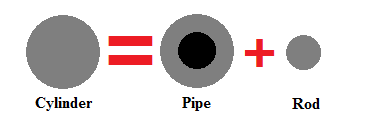# Cylinder $=$ pipe $+$ rod

Consider an iron cylinder, which is divided to extract a rod and a pipe, whose resistances are equal.

Find $\dfrac{R_\text{cylinder}}{R_\text{rod}}$.

Details and Assumptions:

$R_\text{cylinder}=$ Cylinder's radius.

$R_\text{rod}=$ Rod's radius.×